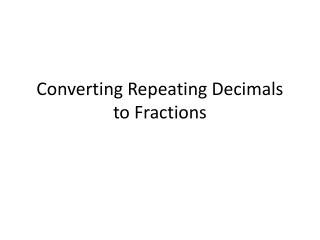DownloadDownload PresentationConverting Repeating Decimals to Fractions

# Converting Repeating Decimals to Fractions

Télécharger la présentation## Converting Repeating Decimals to Fractions

- - - - - - - - - - - - - - - - - - - - - - - - - - - E N D - - - - - - - - - - - - - - - - - - - - - - - - - - -
##### Presentation Transcript

1. Converting Repeating Decimals to Fractions

2. This Gets a Little Complex • As we go through a few examples, I want you to look for patterns.

3. Multiplying by a power of 10 • What happens to my decimal any number every time I multiply by ten? • Start with the number 8.0

5. Repeating Decimals • We need to get the entire portion of the decimal that repeats to the left side of the decimal place • To do this we will multiply each side by a power of ten until this is accomplished

6. Repeating Decimals • Lets look at 0.4 • We will make x = 0.4 • If I multiply both sides by 10 I get: 10x = 4.4 which can break into 10x = 4 + 0.4 x = 0.4 so I can substitute 10x = 4 + (x) • Now I need to get one of the variables isolated • 10x – x = 4 + x – x therefore 9x = 4 x =

7. 0.818181……. • Let x = 0.81 • 100x = 81.81 or 100x = 81 + 0.81 • 100x = 81 + x • 100x – x = 81 + x – x therefore 99x = 81 x =

8. 0.234234234….. x = 0.234 1000x = 234.234 or 1000x = 234 + 0.234 1000x = 234 + x 1000x – x = 234 + x – x therefore 999x = 234 x =

9. Do You See the Pattern? • Can you do this mentally yet? • What is the fractional equivalent of 0.434343….?

10. Why might it be important to be able to convert a repeating decimal to a fraction?

11. Exit • Find the fractional equivalent: • 1) 0.77777….. • 2) 0.527527…… • 3) 0.91269126…….